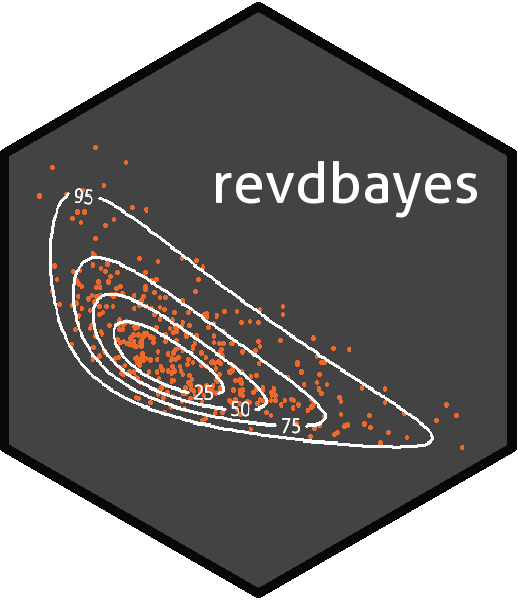# revdbayes### What does revdbayes do?

The `revdbayes` package uses the ratio-of-uniforms method to produce random samples from the posterior distributions that occur in some relatively simple Bayesian extreme value analyses. The functionality of revdbayes is similar to the `evdbayes` package, which uses Markov Chain Monte Carlo (MCMC) methods for posterior simulation. Advantages of the ratio-of-uniforms method over MCMC in this context are that the user is not required to set tuning parameters nor to monitor convergence and a random posterior sample is produced. Use of the Rcpp package enables `revdbayes` to be faster than `evdbayes`. Also provided are functions for making inferences about the extremal index, using the K-gaps model of Suveges and Davison (2010).

### A simple example

The two main functions in `revdbayes` are `set_prior` and `rpost`. `set_prior` sets a prior for extreme value parameters. `rpost` samples from the posterior produced by updating this prior using the likelihood of observed data under an extreme value model. The following code sets a prior for Generalised Extreme Value (GEV) parameters based on a multivariate normal distribution and then simulates a random sample of size 1000 from the posterior distribution based on a dataset of annual maximum sea levels.

``````data(portpirie)
mat <- diag(c(10000, 10000, 100))
pn <- set_prior(prior = "norm", model = "gev", mean = c(0,0,0), cov = mat)
gevp  <- rpost(n = 1000, model = "gev", prior = pn, data = portpirie)
plot(gevp)``````

From version 1.2.0 onwards the faster function `rpost_rcpp` can be used. See the vignette “Faster simulation using revdbayes and Rcpp” for details. The syntax of `rpost` and `post_rcpp` is identical. For example:

``gevp_rcpp  <- rpost_rcpp(n = 1000, model = "gev", prior = pn, data = portpirie)``

### Installation

To get the current released version from CRAN:

``install.packages("revdbayes")``

### Vignettes

See `vignette("revdbayes-vignette", package = "revdbayes")` for an overview of the package and `vignette("revdbayes-using-rcpp-vignette", package = "revdbayes")` for an illustration of the improvements in efficiency produced using the Rcpp package. See `vignette("revdbayes-predictive-vignette", package = "revdbayes")` for an outline of how to use revdbayes to perform posterior predictive extreme value inference. Inference for the extremal index using the K-gaps model is described in `vignette("revdbayes-kgaps-vignette", package = "revdbayes")`